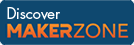Cody

# Problem 29. Nearest Numbers

Created by Cody Team in Community

Given a row vector of numbers, find the indices of the two nearest numbers.

Examples:

` [index1 index2] = nearestNumbers([2 5 3 10 0 -3.1])`
``` index1 =
1
index2 =
3```
` [index1 index2] = nearestNumbers([-40 14 22 17])`
``` index1 =
2
index2 =
4```

Notes

1. The indices should be returned in order such that index2 > index1.
2. There will always be a unique solution.

### Solution Stats

35.26% Correct | 64.74% Incorrect
Last solution submitted on Mar 18, 2019

#### TagsMATLAB and Simulink resources for Arduino, LEGO, and Raspberry Pi